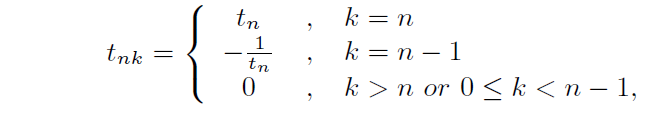##### A Phenomenon in Fibonacci Numbers and Its Generalization

Cherng-tiao Perng, Boyd Coan

Journal of Advances in Mathematics and Computer Science, Page 86-93
DOI: 10.9734/BJMCS/2015/18126

Motivated by the result of Fibonacci numbers for which the ratio of successive terms tends to a limit, which is commonly known as the Golden Ratio, we prove an immediate generalization for a wider class of recurrence sequences. We note that such limiting behavior for ratio of successive terms of general linear recurrence sequences has been well discussed, but still they need to satisfy specic conditions for the limit to exist. Our contribution is that we show that such conditions are indeed satised for the cases we are considering. For an application of our main result, we find a natural way to approximate an algebraic number, which is a zero for some class of polynomial equations, by rational numbers. As recently there seem to be renewed interests on Fibonacci numbers and related recurrence sequences, we hope that our elementary methods and results may shed some light for solving the related problems.

##### Fundamental Domains and Analytic Continuation of General Dirichlet Series

Dorin Ghisa

Journal of Advances in Mathematics and Computer Science, Page 94-111
DOI: 10.9734/BJMCS/2015/18174

Fundamental domains are found for functions defined by general Dirichlet series and, by using basic properties of conformal mappings, the Great Riemann Hypothesis is studied.

##### Numerical and Enumerative Results on Okounkov Bodies

Piotr Pokora

Journal of Advances in Mathematics and Computer Science, Page 112-121
DOI: 10.9734/BJMCS/2015/17733

In this note we focus on three independent problems on Okounkov bodies for projective varieties. The main goal is to present a geometric version of the classical Fujita Approximation Theorem, a Jow-type theorem  and a cardinality formulae for Minkowski bases on a certain class of smooth projective surfaces.

##### Generalized Hyers-Ulam Stability for a Mixed Quadratic - Quartic (QQ) Functional Equation in Quasi-Banach Spaces

K. Balamurugan, M. Arunkumar, P. Ravindiran

Journal of Advances in Mathematics and Computer Science, Page 122-140
DOI: 10.9734/BJMCS/2015/17551

In this paper we establish the general solutions and investigate the Hyers - Ulam stability of the

following functional equation

f(3x + 2y + z) + f(3x + 2y - z) + f(3x - 2y + z) + f(3x - 2y - z)

= 72[f(x + y) + f(x - y)] + 18[f(x + z) + f(x - z)] + 8[f(y + z) + f(y - z)]

+ 24f(2x) + 4f(2y) - 240f(x) - 160f(y) - 48f(z)

in quasi-Banach spaces.

##### On Some Banach Sequence Spaces Derived by a New Band Matrix

Emrah Evren Kara, Merve İlkhan

Journal of Advances in Mathematics and Computer Science, Page 141-159
DOI: 10.9734/BJMCS/2015/17499

In this paper, we de_ne the band matrix T = (tnk) bywhere tn > 0 for all n ∈ N and (tn) ∈ c\c0. By using the matrix T, we introduce the sequence space `p(T) for 1 ≤  p≤. Also, we give some inclusion theorems related to this space and find the ∝-, B-, γ- duals of the space `p(T). Lastly, we investigate the necessary and su_cient conditions for an in_nite matrix to be in the classes (`p(T); λ) or (λ; `p(T)) and give the norm of the operators in B(`p(T); μ (S)), where λ ∈ {`1; c0; c; `} and μ ∈{`1; `1}.

##### Solutions of Some Minimization Problems of a Special Class of Functions

S. T. Obeidat, M. Motawi Khashan

Journal of Advances in Mathematics and Computer Science, Page 160-173
DOI: 10.9734/BJMCS/2015/17254

Solutions of three minimization problems of some classes of functions are obtained. The ndings of the present paper generalize several results related to quantile regression. The new results can be applied in dierent aspects such as weighted l1-norm minimization of curves in, where d is a positive integer, and approximation theory. Some applications of the new results are introduced.

##### Fuzzy Goal Programming Model for Optimal Electricity Allocation

Peter Ayuba

Journal of Advances in Mathematics and Computer Science, Page 174-184
DOI: 10.9734/BJMCS/2015/16498

In this paper, an optimal electricity allocation model (OEAM) was developed for efficient energy consumption. This was done by constructing a weighted maxi sum fuzzy goal programming model where the membership functions (of the objectives) are considered as fuzzy goals and weights are introduced to the over deviational variables. In the achievement function, the weighted deviational variables are minimized. The model optimizes the amount of energy to be delivered to various business units of the XYZ electricity distribution company based on the objective functions and constraints. The results further strengthen our belief that fuzzy goal programming (FGP) models have considerable promise in terms of control, flexibility and real world applicability.